Topic 8 Test- Fractions
Name *
1. Which of the fractions below is equivalent to 3/4? *
2. What number should go in the box to make the fractions equivalent? *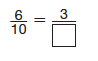3. What is the missing number that makes the fractions equivalent? *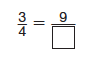4. What are two fractions that represent point M? *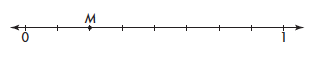5. Luis and Bill raced from their house to the big tree in the backyard, but stopped when their mother called them in. They decided that whoever had gotten farther would win the race. Luis made it 5/8 of the way and Bill made it 3/8 of the way to the tree. Which symbol makes the comparison true for the fractions below? *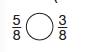6. Karen measured an orange and a tangerine. The orange weighed 3/4 pound. The tangerine weighed 4/8 pound. Which was heavier? *
On your sheet of paper, compare the fractions. You have to find COMMON DENOMINATORS first.
7. The table below shows the different types of juice Pete drank yesterday. Which option below shows the fractions in order for least to greatest? *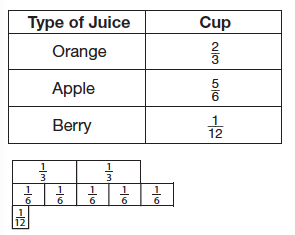8. Which option lists the fractions 5/12, 3/4, and 2/4 in order from least to greatest? *
On your sheets of paper, change the fractions so that they have COMMON DENOMINATORS before you compare.
9. Which of the fractions below is NOT a benchmark fraction? *
10. Which of the fractions below is NOT equivalent to 1/2? *
11. Which fraction below is equal to one whole? *
12. Which fraction below is NOT equal to 3/4? *